# Tarjan’s Algorithm: Strongly Connected Components

Tarjan’s algorithm1, 2 which runs in linear time is an algorithm in Graph Theory for finding the strongly connected components of a directed graph.

## Bridges and Articulation Points

Bridges and Articulation Points are important in Graph Theory because in real-world situations, they often hint weak points, bottlenecks or vulnerabilities in the graph. Therefore, it is important to be able to quickly find and detect where and when they occur.

### Bridge

A Bridge (or cut-edge) in graph theory is any edge in a graph whose removal increases the number of connected components.Lines with the red color are bridges because if you remove any of them, the graph is divided into two components.

### Articulation Point

An Articulation Point (or cut-vertex) is any node in a graph whose removal increases the number of connected components.Nodes marked with orange color are articulation points because if you remove any of them, graph is devided into two components.

## Tarjan’s Algorithm

Tarjan’s Algorithm provides a very effective way to find these bridges and articulation points in linear time. We can explain this algorithm in 3 steps:

### Step 1

Start at any node and do a Depth First Search (DFS) traversal, labeling nodes with an increasing `id` value as you go.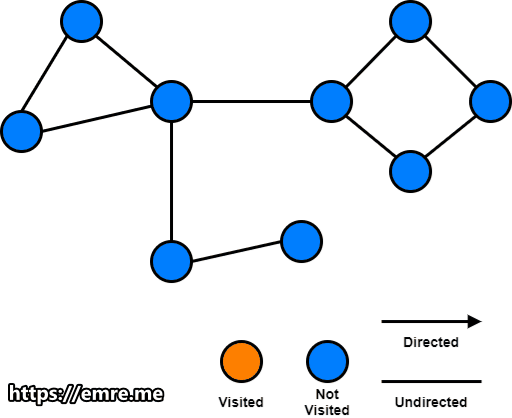### Step 2

Keep track the `id` of each node and the smallest low link value.

Low Link Value of a node is defined as the smallest `id` reachable from that node when doing a Depth First Search (DFS), including itself.

Initially, all low link values can be initialized to the each node `id`.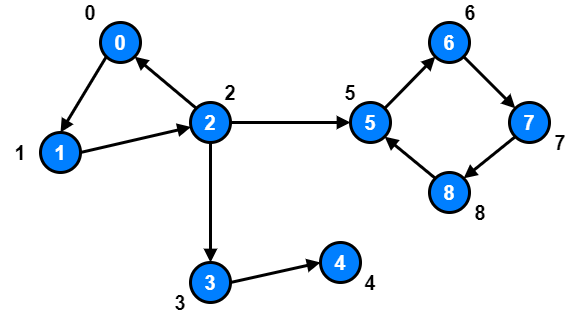If we inspect node 1 and node 2, we will notice that there exist a path going from node 1 and node 2 to node 0.

So, we should update both node 1 and node 2 low link values to 0.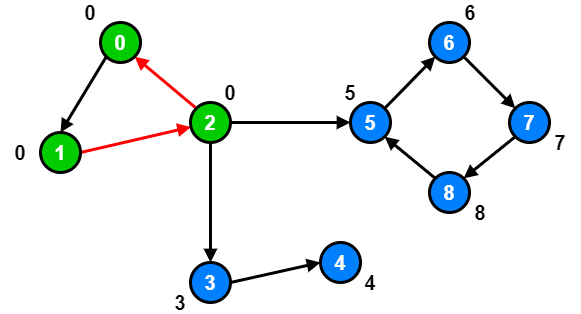However, node 3, node 4 and node 5 are already at their optimal low link value because there are no other node they can reach with a smaller `id`.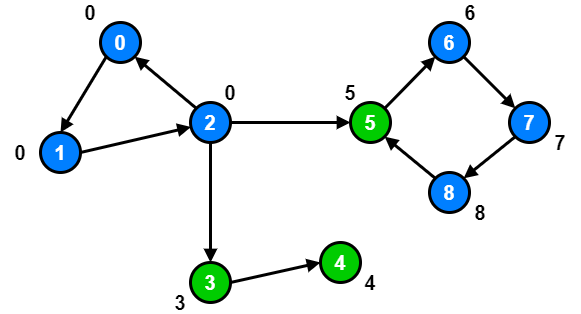For node 6, node 7 and node 8, there is a path from node 6, node 7 and node 8 to node 5.

So, we should update node 6, node 7 and node 8 low link values to 5.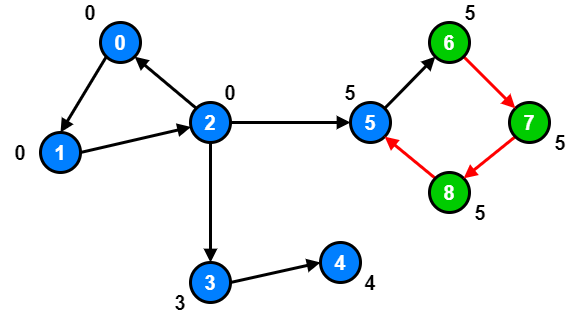### Step 3

During the Depth First Search (DFS), bridges will be found where the `id` of node your edge is coming from is less than the low link value of the node your edge is going to.## Problem: Critical Connections in a Network

LeetCode 1192 - Critical Connections in a Network [hard]

There are `n` servers numbered from 0 to n-1 connected by undirected server-to-server connections forming a network where `connections[i] = [a, b]` represents a connection between servers a and b. Any server can reach any other server directly or indirectly through the network.

A critical connection is a connection that, if removed, will make some server unable to reach some other server.

Return all critical connections in the network in any order.

Example 1: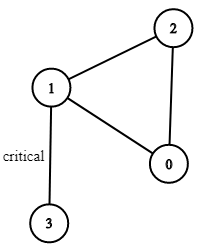``````Input: n = 4, connections = [[0, 1], [1, 2], [2, 0], [1, 3]]
Output: [[1, 3]]
Explanation: [[3, 1]] is also accepted.
``````

Constraints:

• 1 <= `n` <= 105
• `n-1` <= `connections.length` <= 105
• `connections[i] != connections[i]`
• There are no repeated connections.

### Solution with using Tarjan’s Algorithm

``````from collections import defaultdict
from typing import List

class Solution:
def criticalConnections(self, n: int, connections: List[List[int]]) -> List[List[int]]:
def make_graph(connections):
graph = defaultdict(list)
for edge in connections:
a, b = edge
graph[a].append(b)
graph[b].append(a)
return graph

graph = make_graph(connections)

id, node, prev_node = 0, 0, -1  # at first there is no prev_node. we set it to -1
ids = [0 for _ in range(n)]  # tracks ids of nodes
low_links = [0 for _ in range(n)]  # tracks low link value (default value is the index)
visited = [False for _ in range(n)]  # tracks DFS visit status

bridges = []
self.dfs(node, prev_node, bridges, graph, id, visited, ids, low_links)

return bridges

def dfs(self, node, prev_node, bridges, graph, id, visited, ids, low_links):
visited[node] = True
ids[node] = id
id += 1

for next_node in graph[node]:
if next_node == prev_node:
continue
if not visited[next_node]:
self.dfs(next_node, node, bridges, graph, id, visited, ids, low_links)
if ids[node] < low_links[next_node]:  # found the bridge!
bridges.append([node, next_node])
else:
# tried to visit an already visited node, which may have a lower id than the current low link value